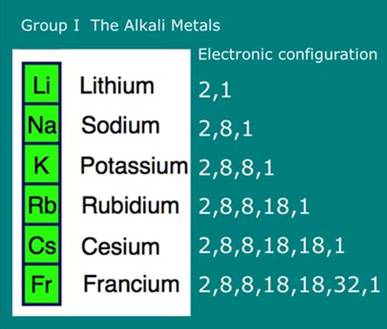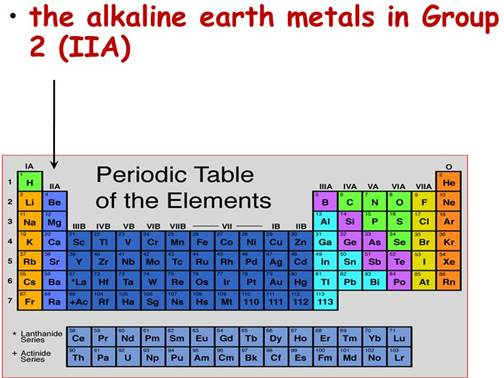SSC BOARD PAPERS IMPORTANT TOPICS COVERED FOR BOARD EXAM 2024

### Question 2. Choose the correct option and rewrite the statement. a. The number of electrons in the outermost shell of alkali metals is......

Question 2.

Choose the correct option and rewrite the statement.

a. The number of electrons in the outermost shell of alkali metals is......

(i) 1 (ii) 2 (iii) 3 (iv) 7

b. Alkaline earth metals have valency 2. This means that their position in the modern periodic table is in .....

(i) Group 2 (ii) Group16

(iii) Period 2 (iv) d-block

c. Molecular formula of the chloride of an element X is XCl. This compound is a solid having high melting point. Which of the following elements be present in the same group as X.

(i) Na (ii) Mg (iii) Al (iv) Si

d. In which block of the modern periodic table are the nonmetals found?

(i) s-block (ii) p-block

(iii) d-block (iv) f-block

a) The number of electrons in the outermost shell of alkali metals is 1.

Explanation:

Alkali metals belong to the group first of the periodic table. The number of electrons in the outermost shell (valence electrons) of all the alkali metals is 1 as shown below:b) Alkaline earth metals have valency 2. This means that their position in the modern periodic table is in Group 2.

Explanation:

It is the electronic configuration of the elements which decides the group in which they are to be placed. The alkaline earth metals have two electrons in the outermost shell. This means that their position in the periodic table is in group 2.Note: Count the number of electrons present in the outermost shell of any element then automatically it will tell you about the group no. of that element.

c) (i)-Na

Explanation:

From the formula XCl, we can see that one atom of X combines with one atom of chlorine(Cl). This means chlorine is combined with the element X who is having valency of one.

In the given elements, Na has one electron in the outermost shell. Hence, Na is the element X as NaCl.

d. (ii)- p-block

Explanation:

The nonmetals are found in the p-block of the periodic table.

The p- block consists of:

(a) Metals

(b) Non-metals

(c) Metalloids (behave as both metals and non-metals)

In p- block, it is seen that the zig- zag line separates the metals from nonmetals in the periodic table.